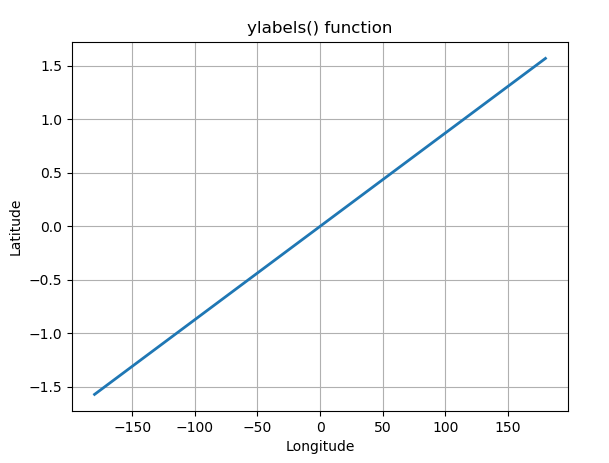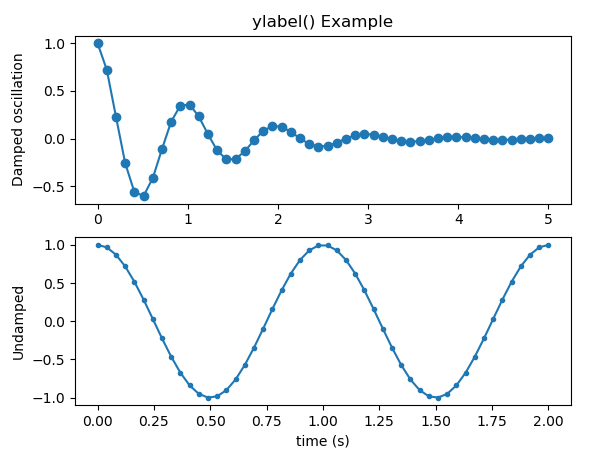# Matplotlib.pyplot.ylabels() in Python

Matplotlib is a library in Python and it is numerical – mathematical extension for NumPy library. Pyplot is a state-based interface to a Matplotlib module which provides a MATLAB-like interface.

## matplotlib.pyplot.ylabel() Function

The ylabel() function in pyplot module of matplotlib library is used to set the label for the x-axis..

Parameters: This method accept the following parameters that are described below:

• ylabel: This parameter is the label text. And contains the string value.
• labelpad: This parameter is used for spacing in points from the axes bounding box including ticks and tick labels and its default value is None.
• **kwargs: This parameter is Text properties that is used to control the appearance of the labels.

Below examples illustrate the matplotlib.pyplot.ylabel() function in matplotlib.pyplot:

Example #1:

 `# Implementation of matplotlib.pyplot.ylabels()  ` `# function ` `   `  `import` `numpy as np ` `import` `matplotlib.pyplot as plt ` `  `  `t ``=` `np.arange(``-``180.0``, ``180.0``, ``0.1``) ` `s ``=` `np.radians(t)``/``2.` `  `  `plt.plot(t, s, ``'-'``, lw ``=` `2``) ` `  `  `plt.xlabel(``'Longitude'``) ` `plt.ylabel(``'Latitude'``) ` `plt.title(``'ylabels() function'``) ` `plt.grid(``True``) ` `  `  `plt.show() `

Output:Example #2:

 `# Implementation of matplotlib.pyplot.ylabels()  ` `# function ` `  `  `import` `numpy as np ` `import` `matplotlib.pyplot as plt ` ` `  `valx1 ``=` `np.linspace(``0.0``, ``5.0``) ` `x2 ``=` `np.linspace(``0.0``, ``2.0``) ` ` `  `valy1 ``=` `np.cos(``2` `*` `np.pi ``*` `valx1) ``*` `np.exp(``-``valx1) ` `y2 ``=` `np.cos(``2` `*` `np.pi ``*` `x2) ` ` `  `plt.subplot(``2``, ``1``, ``1``) ` `plt.plot(valx1, valy1, ``'o-'``) ` `plt.title(``'ylabel() Example'``) ` `plt.ylabel(``'Damped oscillation'``) ` ` `  `plt.subplot(``2``, ``1``, ``2``) ` `plt.plot(x2, y2, ``'.-'``) ` `plt.xlabel(``'time (s)'``) ` `plt.ylabel(``'Undamped'``) ` ` `  `plt.show() `

Output:Attention geek! Strengthen your foundations with the Python Programming Foundation Course and learn the basics.

To begin with, your interview preparations Enhance your Data Structures concepts with the Python DS Course.

My Personal Notes arrow_drop_upSmall things always make you to think big

If you like GeeksforGeeks and would like to contribute, you can also write an article using contribute.geeksforgeeks.org or mail your article to contribute@geeksforgeeks.org. See your article appearing on the GeeksforGeeks main page and help other Geeks.

Please Improve this article if you find anything incorrect by clicking on the "Improve Article" button below.

Article Tags :

Be the First to upvote.

Please write to us at contribute@geeksforgeeks.org to report any issue with the above content.